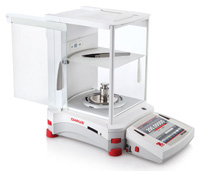MSDSTopics Free Sites FAQ's Regulations Glossary Software Suppliers Books Forum Poll Fun stuff Quiz Store Understand your MSDS with the MS-Demystifier Search ALL our MSDS info

Mass Unit Conversions

### DefinitionGet forklift safety supplies like this training certification card from Safety Emporium.

The term mass is often used interchangeably with "weight". Strictly speaking, this is incorrect because weight varies with the force of gravity and mass does not.

For example, an astronaut would be almost weightless in outer space, but his or her mass would be unchanged from that on Earth. Therefore, one can say that mass is an expression of "how much stuff" something has.

Here is a handy conversion calculator for some common and uncommon mass (weight) terms. Each term is defined in the next section.

 Mass Conversion Calculator(Enter number on the left side; answer appears on the right side) This many: metric tonskilogramsgramscaratsmilligramsmicrogramsnanogramspicogramsfemtogramsattogramszeptogramslong tonsshort tons (tons)poundsouncesdramsgrains Equals metric tons kilogramsgramscarats milligramsmicrogramsnanogramspicogramsfemtogramsattogramszeptogramslong tonsshort tons (tons)poundsouncesdramsgrains

Many of the items you will find on an Safety Data Sheet come in both English (U.S. Customary System) and metric (International System or SI or cgs) units. The metric system has been adopted by almost every country except the United States. Even in the U.S., scientists and technical people use the metric system because of its ease of use.

Metric ton
(t)
A metric ton is equal to 1,000,000 grams = 1,000 kilograms = 2,204 pounds = 1.102 U.S. short tons.
Kilogram
(kg, kilos)
The prefix "kilo" means 1,000, so there are 1,000 grams in a kilogram. One kg = 2.205 pounds. A cube of water 10 centimeters on each side has a mass of 1 kg. For the "official standard" see the links below.
Gram
(g, gm)
A gram is 1/1000th of a kilogram. A gram is equal to the mass of water contained in a cube one cm on each side (one cubic centimeter = 1 cc = 1 cm3 of water weighs 1 gram).
Carat
(c)
Used primarily for gemstones, one carat = 0.2 gram = 0.00705 ounce. There are 5 carats in a gram.
Milligram
(mg, mgs)
The prefix "milli" means 1/1000. Therefore, 1 milligram = 0.001 grams, or 1000 mg = 1 g. 1 mg = 1e-6 kg. 1 mg = 3.52e-5 ounces.
Microgram
(μg, μgs)
The prefix "micro" means 1/1,000,000 = 1e-6. Therefore, 1 microgram = 0.001 milligram = 0.000001 grams, or 1,000,000 μg = 1 g. 1 μg = 1e-9 kg.
Nanogram
(ng, ngs)
The prefix "nano" means 1/1,000,000,000 = 1e-9, so 1,000,000,000 ng = 1e+09 ng = 1 g. 1 ng = 1,000 picograms. 1000 ng = 1 microgram. 1 ng = 1e-12 kg. This unit, along with picogram, femtogram, attogram and zeptogram are unlikely to ever appear on an SDS!
Picogram
(pg, pgs)
The prefix "pico" means 1/1,000,000,000,000 = 1e-12, so 1,000,000,000,000 pg = 1e+12 pg = 1 g. 1 pg = 1,000 femtograms. 1000 pg = 1 nanogram. 1 pg = 1e-15 kg.
Femtogram
(fg, fgs)
The prefix "femto" means 1/1,000,000,000,000,000 = 1e-15, so 1,000,000,000,000,000 fg = 1e+15 pg = 1 g. 1 fg = 1,000 attograms. 1000 fg = 1 picogram. 1 fg = 1e-18 kg.
attogram
(ag, ags)
The prefix "atto" means 1/1,000,000,000,000,000,000 = 1e-18, so 1,000,000,000,000,000,000 ag = 1e+18 ag = 1 g. 1000 ag = 1 femtogram. 1 ag = 1e-21 kg. For comparison, one atom of carbon weighs approximately 2e-23 g so 50,000 atoms of carbon weigh roughly 1 attogram.
Zeptogram
(zg, zgs)
The prefix "zepto" means 1/1,000,000,000,000,000,000,000 = 1e-21, so 1,000,000,000,000,000,000,000 zg = 1e+21 zg = 1 g. 1000 zg = 1 attogram. 1 zg = 1e-24 kg. For comparison, one atom of platinum weighs approximately 3.02e-22 g so 3 atoms of platinum weigh roughly 1 zeptogram. See also atomic mass unit.
Ton
(long ton)
In the U.S., a long ton = 1.12 short tons = 2,240 pounds = 1.016 metric tons.
Ton
(short ton)
In the U.S., a ton is 2,000 pounds = 907.18 kilograms = 0.907 metric tons. This is the "ton" that most Americans use.
Pound
(lb, lbs)
A pound is an English unit of weight. There are 16 ounces or 7,000 grains (a less common unit) or 453.592 grams in a pound.
Ounce
(oz, oz avdp)
An ounce is equal to 437.5 grains, 1/16 of a pound, or 28.350 grams. The system of having 16 ounces in a pound is called the avoirdupois system. Note:
• An ounce is a unit of weight that is not equal to a fluid ounce which is a measure of volume.
• The troy or apothecary weight system has one ounce = 480 grains = 31.103 grams and 12 ounces = 1 pound! That means one avoirdupois ounce is equal to 0.910 troy ounce. Yikes!
Dram
(dr, dr avdp)
Fairly uncommon. There are 256 drams in a pound. 1 dram = 27.34 grains = 1/16 ounces. Caution: apothecary drams and fluid drams are different from the avoirdupois dram (see previous entry).
Grain
(lb, lbs)
Fairly uncommon. There are 480 grains in a troy ounce and 7,000 grains in a pound. One grain = 64.799 milligrams = 0.324 carats.Whether you need micrograms or pounds there's an Ohaus balance to meet your needs at Safety Emporium.

### SDS Relevance

Be very careful to note the units when reading numbers on a Safety Data Sheet. If you ever perform a calculation of any sort, always remember to write the units next to each number in your calculation and make sure that they cancel properly.